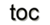Next: 1.3 Topological Toolkit Up: 1. Mathematical Concepts Previous: 1.1 Introduction

# 1.2 Physical Fields and Electromagnetics

This section is dedicated to motivate the subsequent theoretical concepts, introducing a mapping of physical fields to a concise computational framework. One of the fundamental concepts of mathematical physics is that of a field, a spatial distribution of mathematical objects representing a physical quantity . Most of the currently known physical theories build on a common structure  emphasizing the following:

• The existence of a natural association of many physical quantities with geometric objects in space-time.

• The necessity to consider the geometric object to which physical quantities are associated as oriented.

• The existence of two kinds of orientation for these geometric objects.

• The primacy and priority of global physical quantities associated with geometric objects over the corresponding densities.

Therefore, it is necessary to equip the inherently discrete parts of a computer implementation with as much information as possible about the continuous to be projected physical entity. Thereby not only the dimension but also the orientation and information regarding a primary and secondary quantity have to be transfered accordingly.

Here, Maxwell's equations  are briefly introduced, which are extraordinarily important in a special field of scientific computing. It was shown [31,24] that most of the physical theories can be treated similarly, sometimes called the Tonti-diagram, due to the intrinsic nature and structure of the physical concepts. Here, the classic theory of electromagnetics and the macroscopic electric and magnetic phenomena including their interactions are introduced. The following four space-time dependent vector fields are considered:

• the electric field denoted by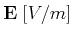• the magnetic field denoted by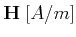• the electric flux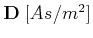• the magnetic flux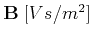The sources of electromagnetic fields are electric charges and currents described by

• the charge density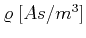• the current density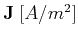where the SI units denote meter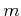, seconds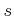, Amperé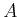, and Volt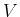. The equations related to electromagnetics in integral formulation read: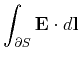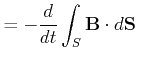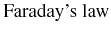(1.1)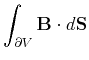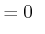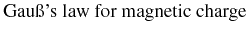(1.2)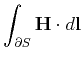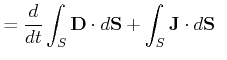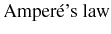(1.3)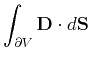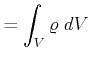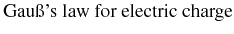(1.4)

whererepresents a volume,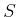a surface, and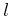a line. Ifis the boundary of a volumein Amperé's law then the boundary ofvanishes (). The field vector can then be eliminated from Amperé's and Gauß's law to obtain a statement about charge conservation: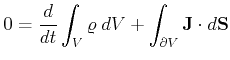(1.5)

If the surfaces and volumes are stationary with respect to the inertial reference frame of field vectors, the local differential versions of the Maxwell equations can be used: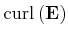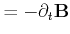(1.6)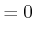(1.7)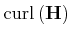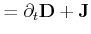(1.8)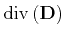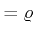(1.9)

The differential versions of Maxwell equations are much less general than the integral laws. The differential laws assume differentiability of the field vectors with respect to spatial coordinates and time. However, there can be discontinuities in fields at material interfaces, so that it is not possible to deduce the proper interface conditions from the differential laws. Therefore, the global integral laws have to be used to derive interface conditions which give relations for the fields at material or media interfaces: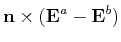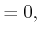(1.10)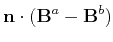(1.11)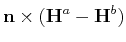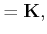(1.12)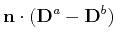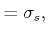(1.13)

where the superscripts refer to values of the field variables corresponding to one of the sides of the interface. It is important to highlight that the source quantities, e.g.,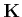, do not really exist, but rather represent a way of modeling current distributions in the limit of zero skin depth .

The integral formulation contains geometric dimensional attributes such as volume or line objects, where the corresponding physical quantities can be calculated. Also, this formulation contains additional topological information, such that an area has to be the surface of a given volume. This information is not given by the differential expression. For the transition to the finite regime of the computer additional information has to be processed to maintain the given structure of physical fields.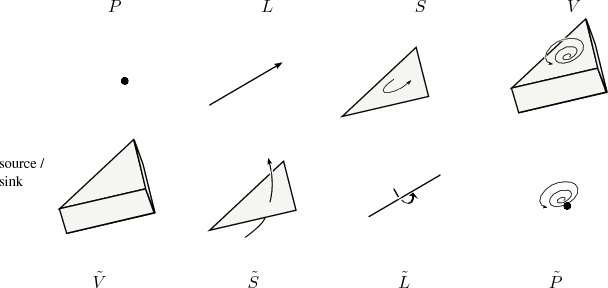Both of these formulations do not contain the important information of the given orientation of the underlying geometrical objects, which is required to assign signs to the given quantities. An example regarding this orientation is the direction of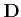through an area, a so-called external orientation, because the direction arrow used is not part of the area itself. Another example is the electric field associated with an internally oriented line segment, which means that the direction arrow is directly associated with the object under consideration. See Figure 1.1 for an overview, where the top line depicts internally oriented objects and the bottom line shows the externally oriented objects with their corresponding dimension.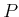states a zero-dimensional object, a point with a source sink orientation,a one-dimensional object (line) with a direction,a two-dimensional object (area) with a circulation direction, and finally rotation direction for a three-dimensional object (volume). The corresponding externally oriented objects are given by. As can be seen, the internal orientation can be described by directions within the given objects, whereas the external orientation requires an additional direction outside the object.

The transition of this modeling step to the regime of the computer demands an additional approximation, the reformulation of the given integral problem in discrete terms with additional orientation information. Based on the given configuration of geometrical objects, the following table associates each physical quantity with the corresponding dimension, orientation, and time attribute. In the following table,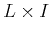states that the lineis actually multiplied by a time interval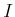, wheremeans discrete time frames.

 dimension physical quantity internally oriented object externally oriented object 1D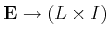line 2D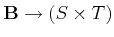area 2D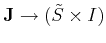area 3D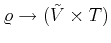volume 1D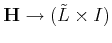line 2D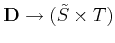area 0D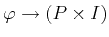point 1D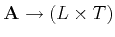line

The necessity of a primary and secondary cell complex can also be easily seen here. The requirement of housing the global physical quantities of a problem implies that both objects with internal orientation and objects with external orientation must be available. Hence, two logically distinct meshes must be defined, one with internal and the other with external orientation.

For general field problems the given Maxwell's equations are not sufficient to determine the electromagnetic field since there are six independent equations in twelve unknowns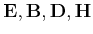. To obtain a consistent equation system, so-called constitutive laws have to be introduced additionally. Then, the given system of equations can be identified by two different classes, whereas the first class represents the structure of a given physical problem .

Definition 1 (Structural law)   Structural laws are conservation, balance, and equilibrium laws.

They state a balance of global quantities whose validity does not depend on metrical or material properties and is therefore invariant for very general transformations. This gives them topological significance and the name topological equation was used  to express the idea of invariance under arbitrary transformations. The second class represents a constitutive law which acts as link between different orientations of a structural law .

Definition 2 (Constitutive law)   Constitutive laws link local field representations.

For the simple case of linear isotropic media, the constitute laws are given by: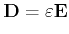(1.14)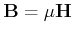(1.15)

Unlike topological laws, constitutive law representations imply the recourse to metrical concepts. This is not apparent in Equation 1.14 because of the use of vectors to represent field quantities, which tends to hide the geometric details of the theory. This leads to the important distinction of topological laws which are intrinsically discrete  and constitutive relations which admit only approximate discrete renderings. For a topological equation the discrete or global version appears as the fundamental one, with the differential statement resulting from it if additional requirements are fulfilled.

The final step towards a complete discrete representation is the association of all given quantities by their corresponding geometrical object. Starting with the global expression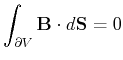(1.16)

which can be written with the complete dimensional information as: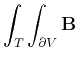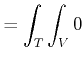(1.17)

This expression can be stated by global quantities only and in a four-dimensional space-time where also a four-dimensional geometrical depiction can be given, where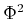represents the associated quantity on a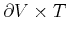object: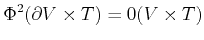(1.18)

It must be mentioned that this expression does not use any material properties and is therefore not an approximation, hence a representation of physical quantities by their intrinsic discrete nature. As an introductory example and a static time frame the expression can be rewritten as: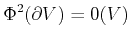(1.19)

By using a, e.g., three-dimensional cell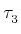, and the corresponding boundary of this cell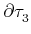, the expression yields: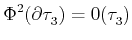(1.20)

To highlight the relation between the cell and the physical quantity, the following pairing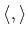can be stated:(1.21)

which lists equivalences of global physical quantities, but still compares to different dimensional quantities, the two-dimensional boundary of a celland the associated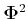, and the three-dimensional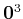on a three-dimensional cell. Another operator, the so-called coboundary operator acts similarly to the boundary operator and can then be used to obtain the following expression: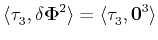(1.22)

As it can be seen, the geometrical dimensions of the given cells correspond to each other, and the final expression states the identity of the two quantities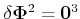.

The following sections now introduce the necessary theoretical concepts to develop a complete and concise framework for this topic. One the one hand, the topological and geometrical concepts are given to handle the underlying discretization of space. On the other hand, concepts required for handling physical quantities and their corresponding operations are introduced. The final goal of this section is to transfer the given physical quantities to the finite regime of the computer without loss of information regarding their dimension, orientation, and pairing with the corresponding geometrical object.Next: 1.3 Topological Toolkit Up: 1. Mathematical Concepts Previous: 1.1 Introduction

R. Heinzl: Concepts for Scientific Computing